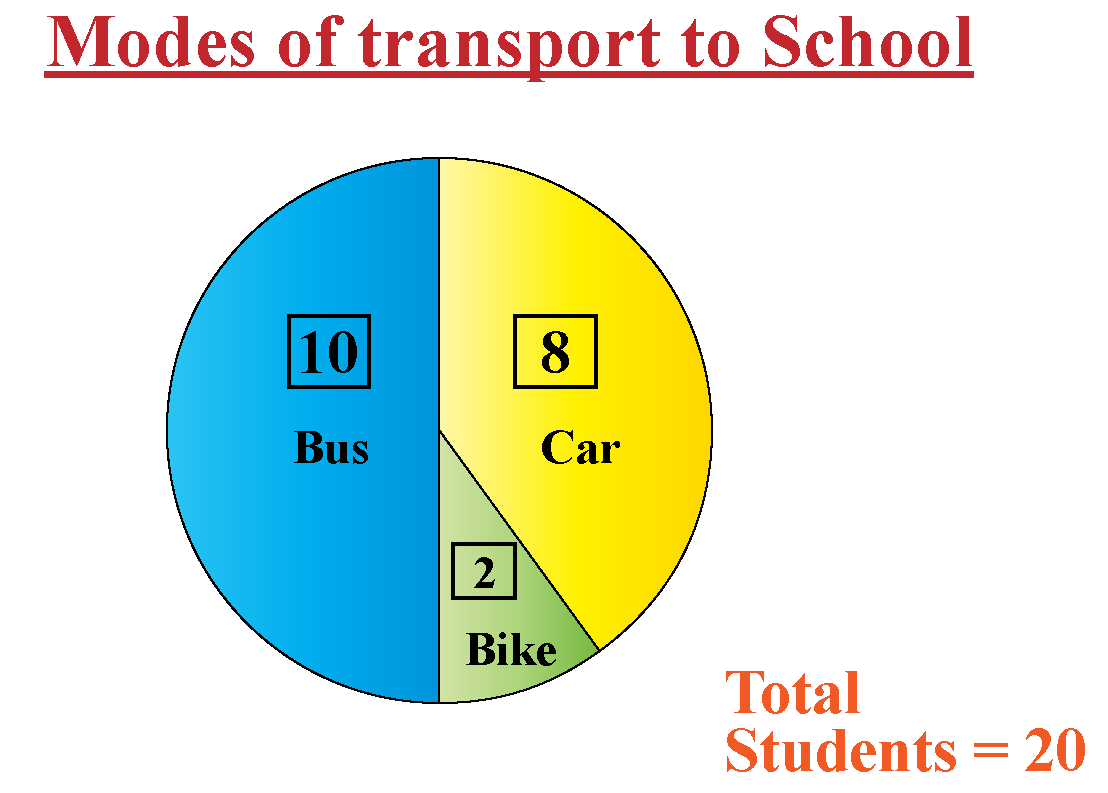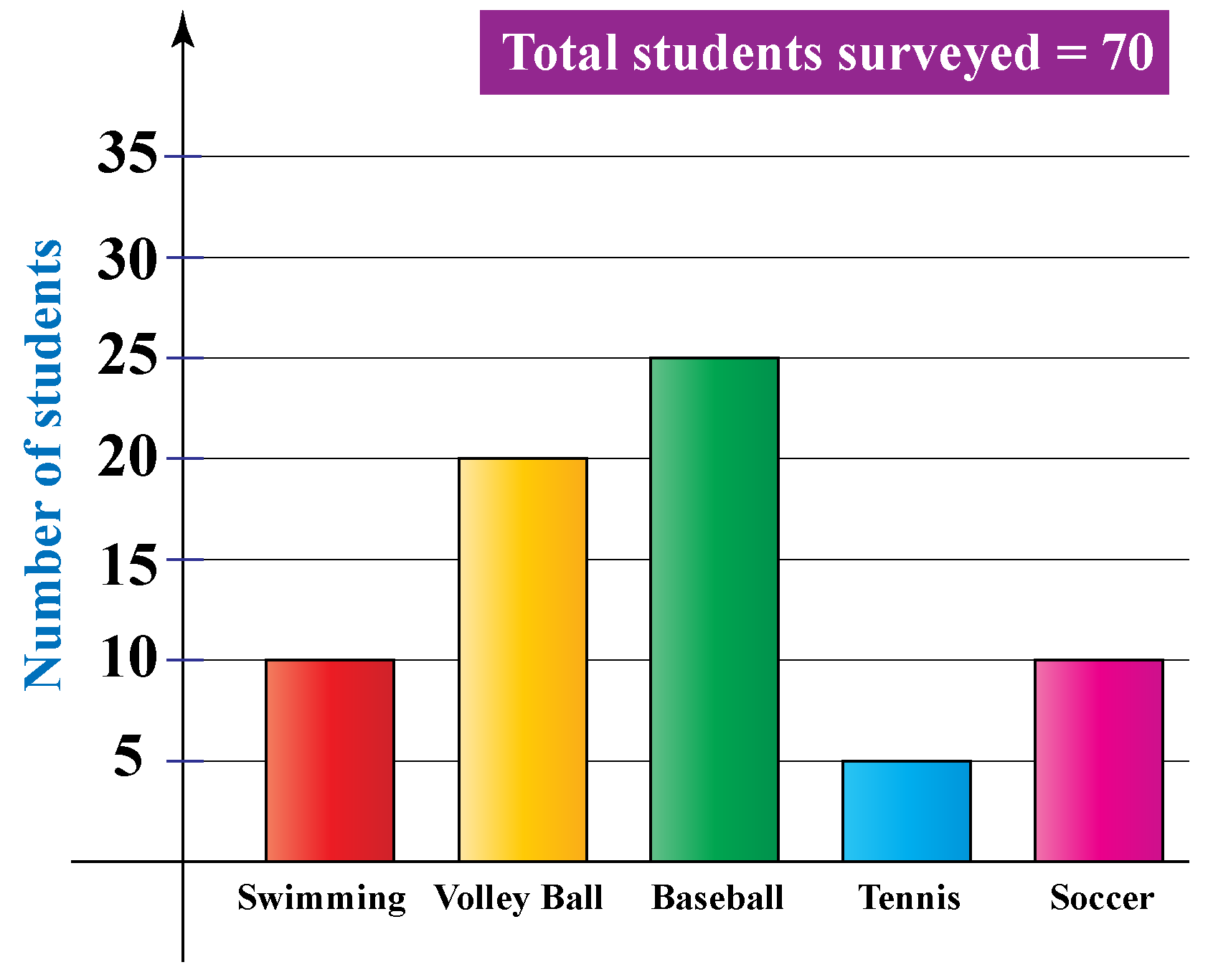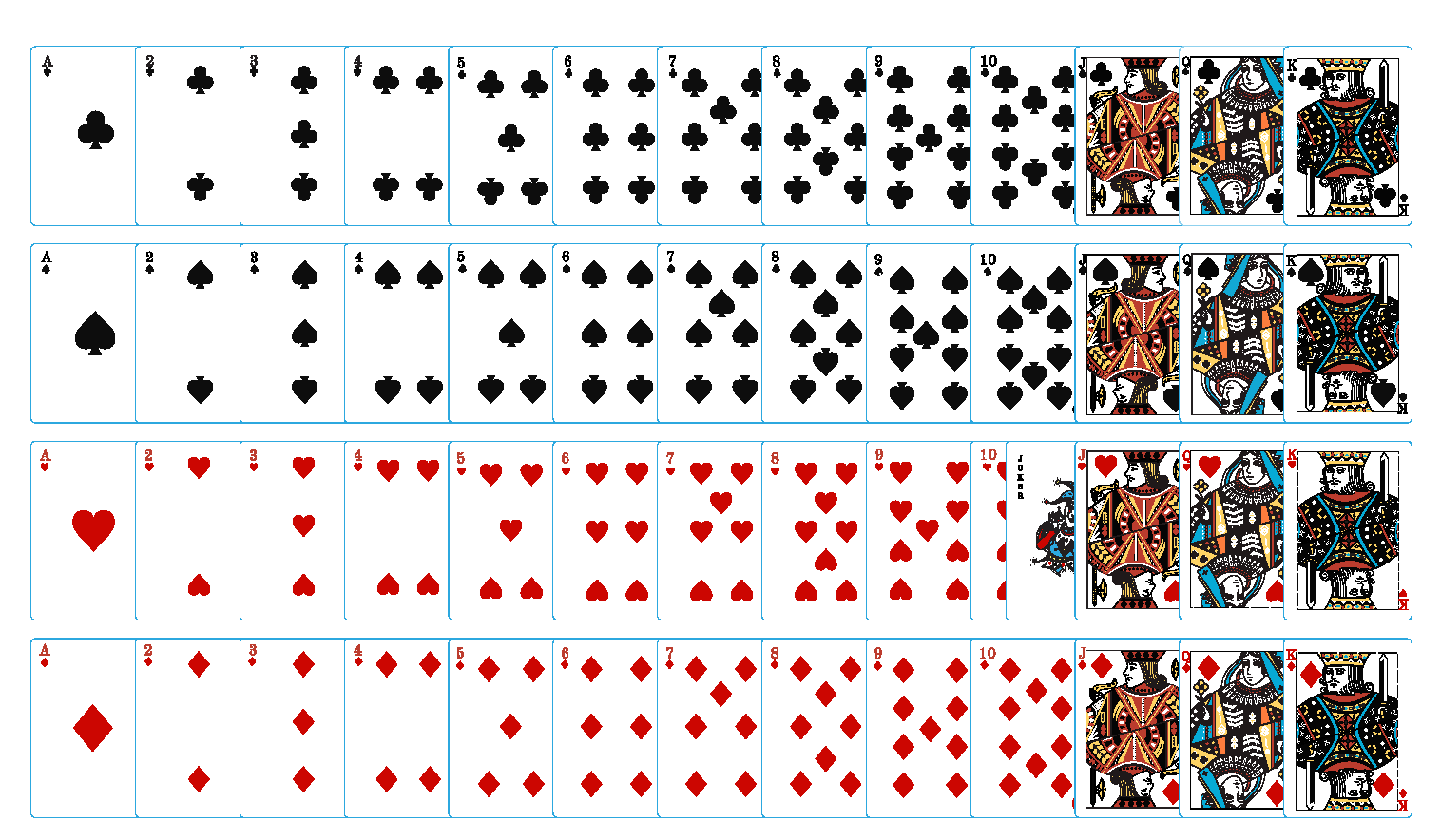# How To Find Relative Frequency

How To Find Relative Frequency

The word 'relative' is used to indicate that an event is being considered in relation or in proportion to something else.

Frequency is a way to measure how often a particular event occurs. Relative frequency, on the other hand, is a way to measure how often a particular event occurs against total occurrences.

#### Example:If 8 out of 20 students travel to school by car, then the frequency for traveling to school by car would be $$8$$

The relative frequency of the same would be:

$\dfrac 8{20} = 40\%$

In this mini-lesson, you will also be acquainted with cumulative frequency, while learning about the relative frequency of data.

## Lesson Plan

 1 What is Meant by Relative Frequency? 2 Thinking Out of the Box! 3 Important Notes on How to Find the Relative Frequency 4 Solved Examples on How to Find the Relative Frequency 5 Interactive Questions on How to Find the Relative Frequency

## What is Meant by Relative Frequency?

#### Relative frequency can be defined as the number of times an event occurs divided by the total number of events occurring in a given scenario.

To calculate the relative frequency two things must be known:

• Number of total events/trials
• Frequency count for a category/subgroup
 $$\text {Relative Frequency}$$=$$\dfrac { Subgroup \: frequency} {Total \:frequency}$$

Example:

Ellie surveys a group of students in her school to learn about their favorite sport. The data collected has been presented below. What will be the relative frequency for volleyball?The total number of students in the data set is found by adding the heights of all the bars
$= 10 + 20 + 25 + 5 + 10 = 70$

The number of students who like volleyball $$= 20$$

So, the relative frequency for the Volleyball will be $$\dfrac {20}{70} = 28.57$$ (to two decimals)

The above frequency can also be expressed simply as a fraction $$\dfrac 27$$

### How to Find the Cumulative Frequency?

In statistics, the frequency of the first-class interval is added to the frequency of the second class, and this sum is added to the third class and so on. Frequencies that are obtained this way are known as cumulative frequency (c.f.).

Example:

Robert is the sales manager of a toy company. On checking his quarterly sales record, he can observe that by the month of April, a total of 83 toy cars were sold.

Month Number of toy cars sold (Frequency) Total number of toy cars sold (Cumulative Frequency)
January 20 20
February 30 20 + 30 = 50
March 15 50 + 15 = 65
April 18 65 + 18 = 83

Note how the last cumulative total will always be equal to the total for all observations since all frequencies will already have been added to the previous total. Here, 83 = 20 + 30 + 15 +18

## What is the General Formula of Relative Frequency?

To find the relative frequency, divide the frequency(f) by the total number of data values (n).

The formula for the relative frequency is given as: $$\dfrac {f}{n}$$

## What is the Difference Between Probability and Relative Frequency?

There is a major difference between relative frequency and probability.

Probability is the measure of an expected event or an event that might occur. It is useful in the cases when each outcome is equally likely. Relative frequency on the contrary measures an actual event that has already occurred.

One can say the probability is theoretical while relative frequency is more practical.

Let's take an example of cards, to get this concept even better.As we all know there are 52 cards, out of which 26 cards are black.

If you had one single chance to randomly pick up a card out of 52 cards, the probability of the black card getting picked will be

\begin{align*} \text {Probability} &= \dfrac{ Number\: of\: black \:cards } {Total\: number\: of \:cards}\\&=\dfrac {26}{52}\\ &=\dfrac12 \\ &=0.5\%\end{align*}

However, to calculate relative frequency, we will need two data sets:

1. Number of times the black cards actually got picked

2. Total trials done for picking a card

So, if out of 10 trials, black cards got picked only 4 times the relative frequency for the black card is

\begin{align*} \text {Relative frequency} &= \dfrac{ Number\: of\: \:times \:black \:cards \:got\: picked} {Total\: number\: of \:trials\:done}\\&=\dfrac {4}{10}\\  &=40\%\end{align*}

## How to Use Relative Frequency Formula?

Let us learn how to use the relative frequency formula to solve the below-given problems.

1. There are 16 students in a class, 6 boys and 10 girls. Find out the frequency as well as the relative frequency of boys?Solution:

Frequency of boys $$\text {(f)}$$ = total number of boys  $$=6$$

For relative frequency, we will use the general formula of the relative frequency $$=\dfrac {f}{n}$$

\begin{align*} \text {Relative frequency} &= \dfrac{ Boys \:count} {Total\: student \:count}\\&=\dfrac {6 }{16}\\ &=37.5\%\end{align*}

We can express relative frequency both as a fraction $$\dfrac {6 }{16}$$ and a percentage $$37.5\%$$Think Tank

A mobile company claims that 95% of its mobile sets do not need repair under the warranty period. 232 handsets were repaired under warranty period out of 5000 handsets sold in 3 months' time.

1. What is the probability that a mobile phone lasts for the warranty period as per the company's claim?

2. What is the relative frequency (in percentage) of the repairs demanded?

## Relative Frequency Calculator• The relative frequency is calculated using the formula:

Relative frequency = number of times an event has occurred / number of trials

• The formula for the relative frequency is given as $$\dfrac {f }{n}$$
• There is a difference between relative frequency and probability.
• The relative frequency, usually expressed as a decimal or percent, is a figure that represents how often an event has already occurred.

## Solved Examples

 Example 1

A cubical die is tossed $$30$$ times and lands $$5$$ times on the number $$6$$. What is the relative frequency of observing the die land on the number $$6$$?

Solution

Given, number of times a die is tossed $$= 30$$

Number of the successful trials of getting number $$6 = 8$$

By the formula, we know,

Relative frequency = Number of positive trial / Total number of trials

$\text f = \dfrac {5}{30} = 16.66\%$

Hence, the relative frequency of observing the die land on the number $$6$$ is $$16.66\%$$

 $$\therefore$$ The relative frequency of observing the die land on the number $$6$$ is $$16.66\%$$
 Example 2

Karen has conducted a survey in her town to know about the dietary habits of people. She records the results as given in the table below.

A) Work out the relative frequency of someone in Karen's town being vegetarian.

B) There are $$1000$$ people in Karen’s town. Find the relative frequency of people in this town who are vegan.

Dietary Habits Frequency
Vegetarian $$218$$
Vegan $$42$$
Mixed diet $$740$$

Solution

A)

Total people = $$1000$$

Number of vegetarians = $$218$$

By the formula, we know,

Relative frequency = Number of vegetarians / Total population

$\text f = \dfrac {218}{1000}= 21.8\%$

Hence, the relative frequency of someone being a vegetarian in this town is $$21.8\%$$

B)

Total people = $$1000$$

Number of vegans = $$42$$

By the formula, we know,

Relative frequency = Number of vegans / Total population

$\text f = \dfrac {42}{1000}= 4.2\%$

Hence, the relative frequency of vegans in the town is $$4.2\%$$

 $$\therefore$$ The relative frequency of vegetarians and vegans in the town is $$21.8\%$$ and $$4.2\%$$ respectively.
 Example 3

Anna has a packet containing $$20$$ candies. Her favorites are the yellow ones and the red ones. The table below shows the frequency of each different candy selected as she picked all $$20$$ sweets one by one and finished them all.

Candy color Yellow Red Green Brown
Frequency $$6$$ $$6$$ $$3$$ $$5$$

A) What is the relative frequency of the picked candy to be one of her favorites?

B) What is the relative frequency for the brown candy

Solution

Relative frequency = number of times an event has occurred / number of trials

A) Relative frequency of the picked candy to be one of her favorites

$$=\dfrac { Frequency\hspace{2mm} of\hspace{2mm} yellow + Frequency\hspace{2mm} of\hspace{2mm} red\hspace{2mm} candy} {20} = \dfrac {12}{20} = 60\%$$

B) Relative frequency of the brown candy

$$=\dfrac { Frequency\hspace{2mm}of \hspace{2mm} brown \hspace{2mm}candy} {20}$$ = $$\dfrac {5}{20}$$ = $$25\%$$

 $$\therefore$$ The relative frequency for Anna's favorite candy is $$60\%$$ and for the brown candy it is $$25\%$$
 Example 4

A tire company runs a durability test on its batch of $$600$$ tires. The test result concludes that $$560$$ out of a batch of $$600$$ tires will withstand $$10,000$$ miles of normal wear. Find the relative frequency of tires that will not last $$10,000$$ miles.Solution

Relative frequency = number of times an event has occurred / number of trials

$$40$$ tires failed the durability test.

The relative frequency of the $$40$$ tires

$=\dfrac{40}{600}\\=6.66\%$

 $$\therefore$$ The relative frequency of tires that will not last $$10,000$$ miles is $$6.66\%$$
 Example 5

A car dealer wants to calculate the total sales for the past month and wants to know the monthly sales in percentage after weeks 1, 2, 3, and 4. Create a relative cumulative frequency table and present the information that the dealer needs.

Week No. of Cars Sold
1 10
2 17
3 14
4 11

Solution

First total up the sales for the entire month:

10 + 17 + 14 + 11 = 52 cars

Then find the relative frequencies for each week by dividing the number of cars sold that week by the total:

• The relative frequency for the first week is: 10/52 = 0.19
• The relative frequency for the second week is: 17/52 = 0.33
• The relative frequency for the third week is: 14/52 = 0.27
• The relative frequency for the fourth week is: 11/52 = 0.21

To find the relative cumulative frequencies, start with the frequency for week 1, and for each successive week, total all of the previous frequencies

Week Cars sold Relative Frequency Relative Cumulative Frequency
1 10 0.19 0.19
2 17 0.33 0.19 + 0.33 = 0.52
3 14 0.27 0.52 + 0.27 = 0.79
4 11 0.21 0.79 + 0.21 = 1

*Note that the first relative cumulative frequency is always the same as the first relative frequency, and the last relative cumulative frequency is always equal to 1.

## Interactive Questions

Here are a few activities for you to practice. Select/Type your answer and click the "Check Answer" button to see the result.

## Let's Summarize

This mini-lesson targeted the fascinating concept of relative frequency. The math journey around relative frequency starts with what a student already knows, and goes on to creatively crafting a fresh concept in the young minds. Done in a way that not only it is relatable and easy to grasp, but also will stay with them forever. Here lies the magic with Cuemath.

At Cuemath, our team of math experts is dedicated to making learning fun for our favorite readers, the students!

Through an interactive and engaging learning-teaching-learning approach, the teachers explore all angles of a topic.

Be it worksheets, online classes, doubt sessions, or any other form of relation, it’s the logical thinking and smart learning approach that we, at Cuemath, believe in.

## 1. What is the relative frequency table?

A frequency table presents how often a particular event occurs in a tabulated manner.

## 2. What is the difference between frequency and relative frequency?

Frequency is a way to measure how often a particular event occurs. Relative frequency on the other hand is a way to measure how often a particular event occurs against total occurrences.

## 3. What are conditional relative frequencies?

Conditional relative frequency is one of the types of relative frequency. It can be obtained from a two-way frequency table.

It tells us how many members of a given group have a particular characteristic.

More Important Topics
More Important Topics
Learn from the best math teachers and top your exams

• Live one on one classroom and doubt clearing
• Practice worksheets in and after class for conceptual clarity
• Personalized curriculum to keep up with school